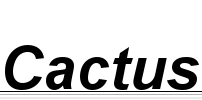## Unary arithmetics is even slower than you'd expect

19 April 2010 (haskell programming math agda correctness)

My coworker Encsé posted a challange use the 9-digit number problem to demonstrate interesting programming techniques. So I sat down and wrote my first practical program using dependant types, in Agda. I've been playing around with Agda previously, but this seemed like a good opportunity to try to write an actual, self-contained, provably correct program with it.

I'm not going to get into details now about the resulting Agda code, because I'm planning to present it in detail in a later post. In its current form, my program is formally proven to produce only good nine-digit numbers; the only property that still needs proving is that it finds all of them.

But the sad surprise came when I tried to actually run it. It was unbearably slow to just get to all possible combinations for the first three digits. I'm talking about 12 minutes just to list them from 123 to 423 (at which point I killed the process). For comparison, the following Haskell program, which is an implementation of the same naïve algorithm, finds the (unique) solution in 4 milliseconds:

```import Control.Monad.List

fromDigits = foldr shift 0
where shift d s = 10 * s + d

p `divides` q = q `mod` p == 0

encse :: [Int]
encse = map fromDigits \$ encse' 0 []
where encse' 9 ds = return ds
encse' n ds = do d <- [1..9]
let ds' = d:ds
n' = n + 1
guard \$ not (d `elem` ds)
guard \$ n' `divides` fromDigits ds'
encse' n' ds'```

### So where's that slowdown coming from?

The first intuition would be that the code generated by Agda is slow because in parallel to the actual computation, it is also evaluating all kinds of proofs. But the proofs exist only in the world of types, so they shouldn't matter once the program is compiled.

The real answer is that calculating in unary representation is slow. Very, very slow. Even slower than you'd imagine.

Of course, Agda uses Peano arithmetic because it's easy to argue about function properties in unary. But it comes with a huge cost in performance: operations have run-times proportional to their input itself, not its order of magnitude. Even converting an unary number to a human-readable string becomes an expensive operation, as the following Agda program demonstrates:

```module ShowNat-Native where

open import Data.Nat
open import Data.Nat.Show
open import Data.List

open import IO
import Data.Colist

numbersBelow : ℕ → List ℕ
numbersBelow zero     = []
numbersBelow (suc n)  = numbersBelow n ++ [ n ]

main = run (mapM′ putStrLn (Data.Colist.fromList  (Data.List.map show (numbersBelow 500))))```

If you try out this program yourself (I've been using version 0.2 of the Agda standard library for these tests), you can see that the numbers appear increasingly slowly on the output. On my machine, it takes approximately five and a half minutes just to display the first 500 natural numbers.

### Can we do something about it?

In fear of becoming the laughingstock of Encsé for writing an elaborate, theoretically sound program that cannot be executed, I've explored pushing down operations into Haskell.

Of course, the more you push into Haskell, the less you can formally prove about your Agda program. For some functions, there's no real loss; for example, the signature of Data.Nat.Show.show is simply ℕ → String, which (apart from the requirement of finiteness) we can easily emulate in Haskell.

To give you an idea of the possible speedup, modifying the code above to use an implementation of show written in Haskell, the runtime now becomes 24 milliseconds:

```module ShowNat-Foreign where

open import Data.Nat
open import Data.ForeignNat.Show
open import Data.List

open import IO
import Data.Colist

numbersBelow : ℕ → List ℕ
numbersBelow zero     = []
numbersBelow (suc n)  = numbersBelow n ++ [ n ]

main = run (mapM′ putStrLn∞ (Data.Colist.fromList  (Data.List.map show (numbersBelow 500))))```

The devil's in the details, of course: in this case, in the ForeignNat library. I've uploaded a browsable snapshot here, and there's a nice HTML version here. If you want to try it out, I suggest getting it from the Git repository using the following command:

git clone git://gergo.erdi.hu/agda/foreign-nat

It includes not just Data.ForeignNat.Show used above, but also Data.ForeignNat.Divisibility, which calculates the remainder in Haskell, using Haskell's Integer type to speed things up. Of course, deciding divisibility also means that it needs to produce a proof of divisibility, so there's more plumbing required on the Agda side.

I haven't yet modified my 9-digit number searcher program to use these foreign implementations, but I'm looking forward to seeing if implementing these speedups finally permit running the damn program.